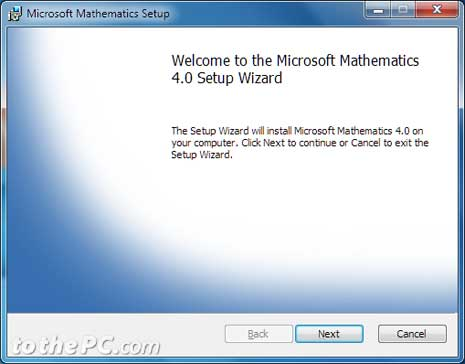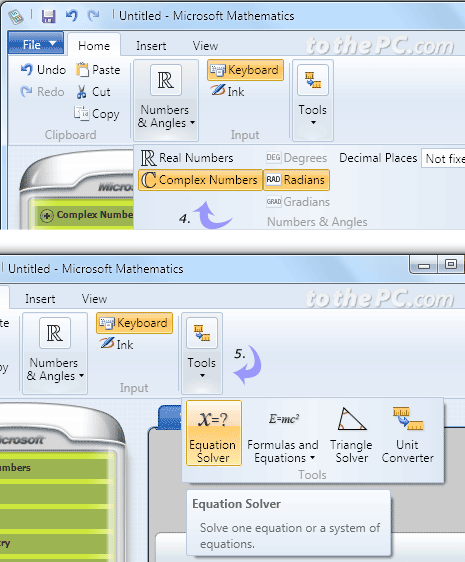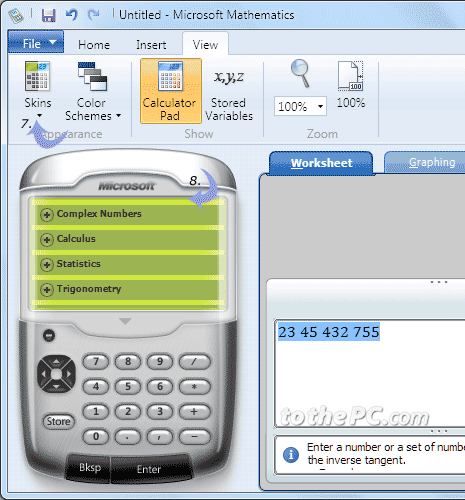# Solve Math equations with Microsoft Mathematics

Are you confused while trying to solve complex pre-algebra, algebra, trigonometry, physics, chemistry or calculus problems? You can easily get going with Math equations and formulas using Microsoft Mathematics program. It is a free software program from Microsoft for Windows users to solve Math equations step by step with better understanding. Simple interface help solve complex Math questions quickly without too much effort.

1. Open download page for Microsoft Mathematics program. If you are using 64bit Windows, then click download button next to MSetup_x64.exe option. While 32bit Windows users need to click download button next to MSetup_x86.exe option. [Is my Windows 32 or 64 bit].2. Once download is complete, run the setup wizard. Click next button to start installation of Microsoft Mathematics software program.

3. After installation, launch Microsoft Mathematics program from Start menu.

4. Under Home tab you can work with Real numbers, Complex numbers, radians, decimal places and so on.5. You can also access tools like equation solver, formulas, triangle solver and unit converter applications.

6. Under insert tab you can insert matrix equation, different variables and data sets.

7. Under View tab you can customize look of Microsoft Mathematics program by selecting different skins and color schemes.8. Access left tab menu for specific math problems of calculus, statistics, trigonometry, liner algebra and more.

9. You can easily find way through looking at examples displayed at bottom of the application window. Once you are done with calculations, you can export contents to Microsoft Word file or save it in Microsoft Mathematics Worksheet .gcw format.

## Perform Math calculations online

1. You can use Wolfram online tool to find answer to Math integration problems.

2. There are number of online resources to calculate LCM / HCF of numbers, find prime numbers out of given set of numbers and so on.

SHARE